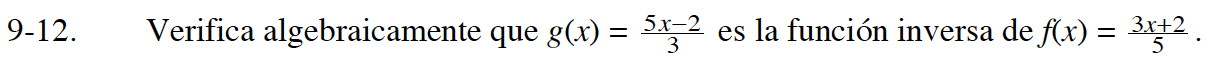### Home > CCA2S > Chapter 9 > Lesson 9.1.1 > Problem9-12

9-12.Sustituye la f(x) en g(x). Simplifica.

$g(f(x))=\frac{5\left(\frac{3x+2}{5}\right)-2}{3}=x$